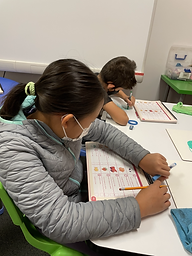## Mr. Jake

### Target 1​

###### Lesson Type:

New

Data Probability

:

Ratio

Use ratio understandings to identify a unit rate.

###### 1:

Describe a unit rate in the form a:b and a/b.

###### 2:

Solve unit rate problems for unit price.

###### 3:

Define rate, unit rate, and unit price.

###### 4:

Calculate unit rates.

Simplify ratios.

6th

###### Vocabulary:

Ratios, Comparisons, Unit Rate, Unit Price

Activities:

Students used random objects and scales to create ratios (ex.: 20 cubes to 100 pom-poms).

Students simplified ratios so that they corresponded to one item (ex.: 1 cube to 5 pom-poms).

Students calculated unit prices to find the best deal (ex.: \$20 for 5 apples or \$15 for 3 apples).

Students differentiated unit rate and unit price.### Home Exploration

###### Guiding Questions:## Absent Students:

### Target 2

:

###### 1:

Recognize that “rounding” is the identification of the multiple of ten that is the closest to the given number (10, 100, 1000, 1/10, 1/100, 1/,000, etc.).

###### 2:

Visually represent the rounding of decimals on a number line.

###### 3:

Round numbers with fractional parts, using the same value concepts used to round whole numbers.

6th

###### Vocabulary:

Round Up, Round Down, Nearest Tenth/Hundredth, Whole Number, Decimals

Activities:

Students reviewed how to round to the nearest whole number, tenth, and hundredth.

Students played a game where they threw a bean bag at a wall full of Post-Its, which had decimals. They had to round the decimals to the nearest whole number and the nearest tenth. Whoever collected the most Post-Its won.### Home Exploration

###### Guiding Questions:### Target 3

:

###### Vocabulary:

Activities:### Home Exploration